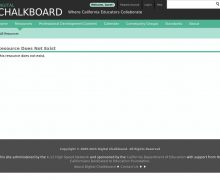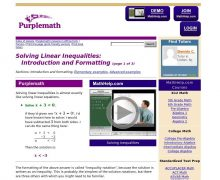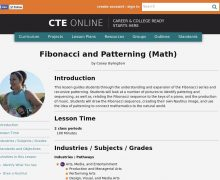Recent Uploads
• # Math: Algebra

Browse All 6 ResourcesIn this tutorial, "Linear functions of the form f(x) = a x + b and the properties of their graphs are explored interactively using an applet." The applet allows students to manipul...From Elizabeth Stapel at Purplemath, this module helps students understand how to solve linear inequalities with an introduction to the topic using both inequality notation and a g...This lesson guides students through the understanding and expansion of the Fibonacci series and recursive patterning. Students will look at a number of pictures to identify patteri...In this tutorial, "Linear functions of the form f(x) = a x + b and the properties of their graphs are explored interactively using an applet." The applet allows students to manipul...From Elizabeth Stapel at Purplemath, this module helps students understand how to solve linear inequalities with an introduction to the topic using both inequality notation and a g...This lesson guides students through the understanding and expansion of the Fibonacci series and recursive patterning. Students will look at a number of pictures to identify patteri...